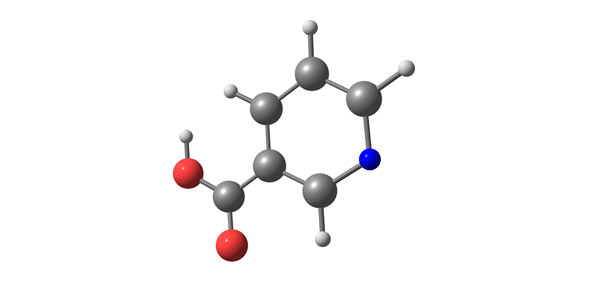Atom Test, Physical Science Test/ Review For Midterm

5 Questions | Total Attempts: 919SettingsThe study of science is an endlessly complex one, but in this quiz our aim is to help teach you some of the basics of a number of the most important topics in the whole study – including atoms and the periodic table. What can you tell us about all of it? Let’s find out!

• 1.
What is a Hypothesis?
• A.

A prediction

• B.

An educated guess

• C.

A guess in If...Then...Because format

• D.

Something in If...Then...Because format

• E.

A prediction in If...Then...Because format

• 2.
What plus what equals the atomic mass?
• A.

N+p

• B.

E+p

• C.

N+e

• D.

Z+a

• E.

Z+p

• 3.
What is the symbol for the atomic mass?
• A.

A

• B.

S

• C.

O

• D.

M

• E.

Z

• 4.
What are isotopes?
• A.

The same amount of electrons but different neutrons

• B.

The same amount of neutrons but different electrons

• C.

The same amount of neutrons but different protons

• D.

The same amount of electrons but different protons

• E.

The same amount of protons but different neutrons

• 5.
What were the four different types of metals?
• A.

Wood, nonwood, tin, iron

• B.

Gold,platnium, rubium, silver

• C.

Metal, nonmetal, transitions, metalliods

• D.

Metal, non-metals, tin, metalliods

• E.

Metal, nonmetals, transitional and metalliods

Related TopicsBack to top